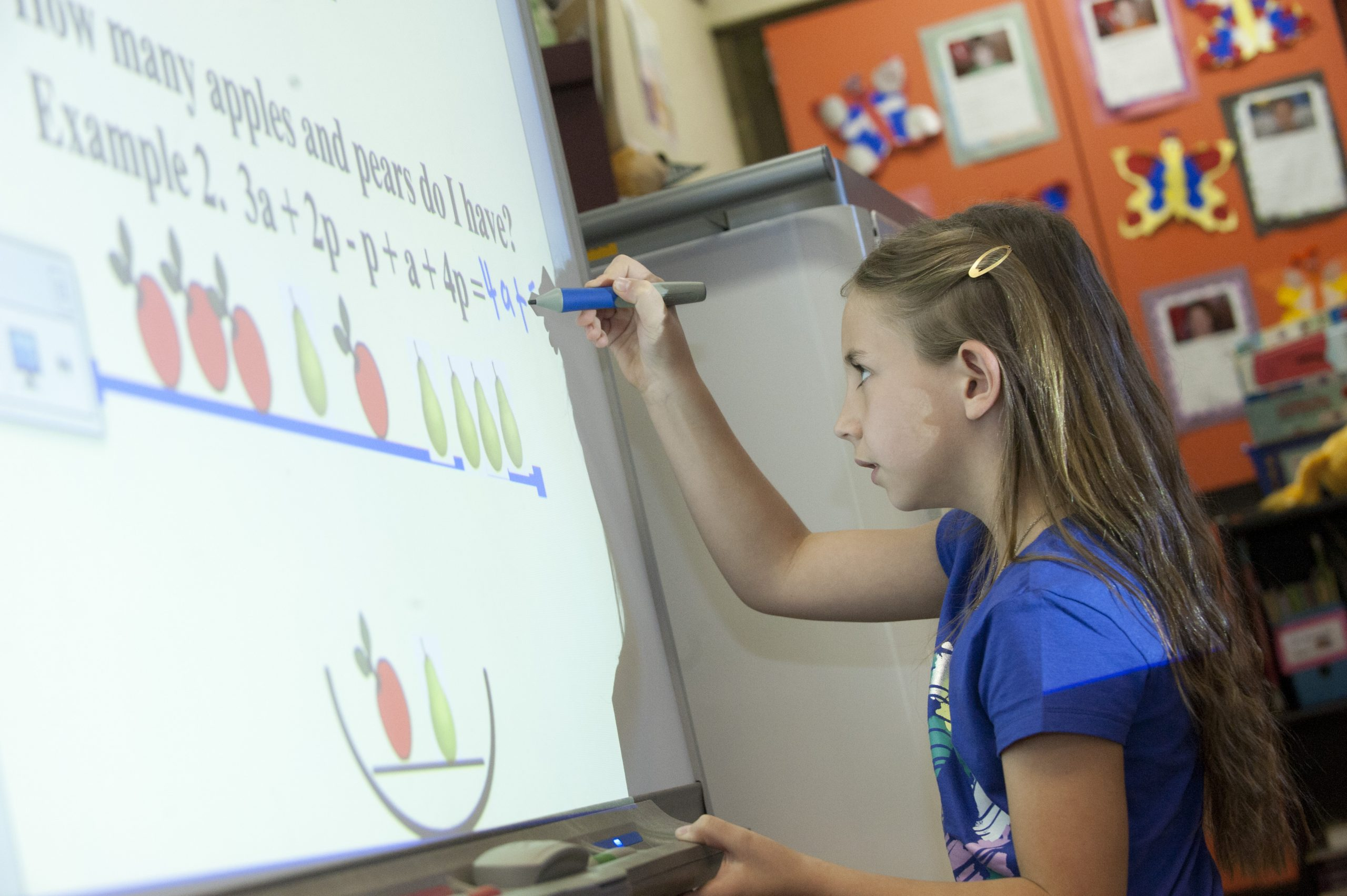# Early Algebra for the SMART Board

Leave a Review

\$25.00

This SMART Board application is intended for teachers of gifted students in grades 1 to 3. Students determine the unknown value in addition and subtraction equations, and they evaluate expressions using objects and drawings. This application consists of eight lessons with 10 examples per lesson. A video overview is included illustrating sample problems for each lesson. A set of worksheets, one per lesson, and an answer key are also included. REQUIRES THE USE OF THE SMART BOARD and SMART NOTEBOOK SOFTWARE 10.0 OR LATER.

SKU: EA-SB-01

## Early Algebra for the SMART Board

Each license includes the following BONUS: An Instructional Video Manual, a set of worksheets, and an answer key.

• Evaluate 1: 2a + 3p if a = 5 and p = 10.
• Example 2: Find the unknown value in 3a – a + 2p – p = 2a + ___
• Example 3: 4 + __ + 5 = 10 – 2 + 3